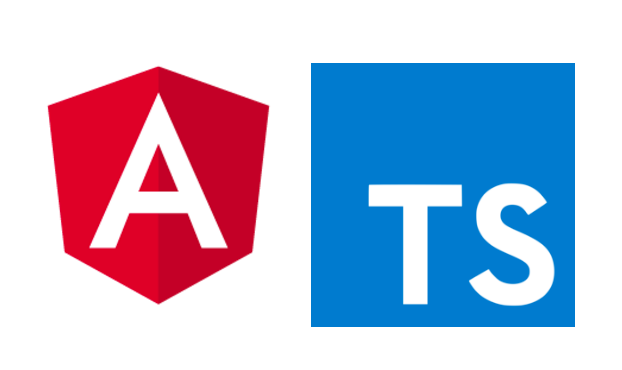## TypeScript

### 緣起

TS是為了解決 JS諸多的問題，例如資料型別(typing)、名稱空間(namescpace)以及零碎的眉眉角角，而且由於當初設計 JS的階段過於倉促，加上沒有先例可以參考(第一個同時兼具函數程式和物件導向程式的語言)，總總原因讓大家對 JS不太滿意，於是就有很多改善 JS的語言誕生，它們都希望減輕 JS開發人員的負擔，改用一些結構良好或是更輕鬆的語言來開發應用程式(Script#、CoffeeScript)，再透過各自的編譯器來產出 JS程式碼， TS也是其中一個。

### 語法

#### const & let

const cannotChange = 9; // 只要改變這個常數，程式會出錯
let canChange = [{'a': '1'}, {'b': '2'}]; //在不改變宣告的資料型別的情況下，可以改變這個參數


var雖然還是能用，但能不用盡量不要用，越明確的規範對程式幫助越好。

#### 資料型別(typing)

//Number
let decimal: number = 6;
let hex: number = 0xf00d;
let binary: number = 0b1010;
let octal: number = 0o744;
//Stirng
let person: string = "Mike"; //可以用 ""
let age: number = 37;
let sentence: string = Oh, ${person} is${age} years old.; //也可以用 ${} //上面等於 "Oh, " + person + " is " + age + " years old." //Array let list: number[] = [1, 2, 3]; //Tuple let x: [string, number]; // Array中包含不同型別的變數用 Tuple x = ["hello", 10]; // OK x = [10, "hello"]; // Error x = true // Error 往後的變數只能是一開始設定的 string 或 number  #### 型別註釋(Type annotations) 以往在 JS中函數的參數是不需要宣告參數的型別。 ##### JS: function greeter(person) { return "Hello, " + person; }  但是這樣沒人知道 person是甚麼，到底是物件還是字串？ ##### TS: function greeter(person: string) { return "Hello, " + person; }  所以 TS強制要求參數要註釋型別 person: string這樣一看就知道是字串，當專案越做越大，函數呼叫函數又在呼叫下個函數，參數滿天飛的時候，才不會搞錯資料型別，又 debug半天才發現是資料給錯！ 註釋也可以放入指定型態的物件，就是接下來的 Interface。 #### Interfaces interface 讓參數為物件時，有更明確的結構型態，而不是只是丟個參數表示為一個物件。 簡單的範例應該可以讓大家懂 interface 的概念。 interface Person { firstName: string; lastName: string; } function greeter(person: Person) { return "Hello, " + person.firstName + " " + person.lastName; } var user = { firstName: "Tiger", lastName: "Liu" }; console.log(greeter(user));  #### 類別(Classes) 以往在 ES5 的 JS 是沒辦法像 C++、JAVA那樣直接宣告類別的，JS開發者當然還是能透過一些技巧達到類似效果，但就比較麻煩也很不直觀。而 ES6 版本之後 JS 加入類別的語法糖，TS 中就能用相同的方式宣告類別，編譯成 JS後其實就是以前我們用來模擬類別的技巧啦！XD class Student { fullName: string; constructor(public firstName, public middleInitial, public lastName) { this.fullName = ${firstName} ${middleInitial}${lastName};
}
}
interface Person {
firstName: string;
lastName: string;
}
function greeter(person : Person) {
return Hello, ${person.firstName}${person.lastName};
}
let user = new Student("Jane", "M.", "User");
console.log(greeter(user));


#### 靜態成員

class Human {
static hands: number = 2;
static legs: number = 2;
}


#### 繼承

class Woman extends Human {
static gender: string = 'female';
}


#### Interface 進階

interface 也可以用來強制類別符合約束

interface Shape {
area(): number;
}
class Circle implements Shape {
}
area(): number {
}
}


interface 也可以繼承其他的 interface

interface Shape {
area(): number;
}
interface Color {
RGB: string;
}
interface Thing extends Color, Shape {
}


#### 迭代

let list = [4, 5, 6];
for (let i in list) {
console.log(i); // "0", "1", "2",
}
for (let i of list) {
console.log(i); // "4", "5", "6"
}


let ... of ... 的操作其實長這樣

let list = [4, 5, 6];
for (let _i = 0; _i < list.length; _i++) {
var num = list[_i];
console.log(num);
}